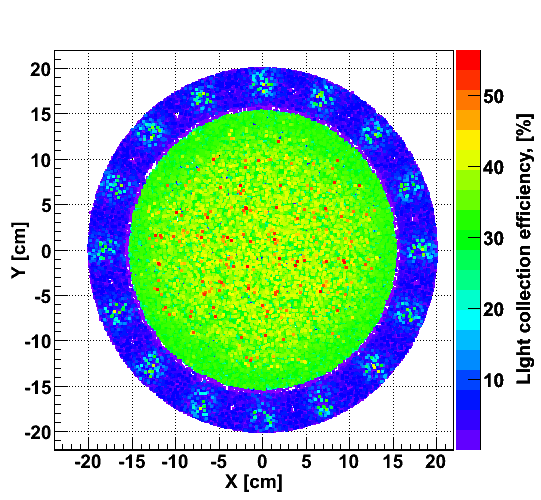# TH2 or TH3? Binning problem

Hello.

I am trying to create a scatter plot with COLZ option, which shows three variables (like shown in the attached figure, here it is X and Y coordinates and Light Collection Efficiency).

To make this plot I create a TH2F:
TH2F *hXYhits = new TH2F(“hXYhits”,"", nbins_X, X_min, X_max, nbins_Y, Y_min, Y_max);

and fill it like:
t1->Draw(“Y:X:LCE>>hXYhits”, “”, “COLZ”);

The problem is that in this case I plot a marker at each XY point, but the binning of the histogram is ignored.

I tried TH3 also, but then I get 3D plot, and this is not what I need.```t1->Draw("Y:X:LCE>>hXYhits", "", "COLZ"); ```do

```t1->Draw("Y:X>>hXYhits", "LCE", "COLZ"); ```
Rene

Rene, thank you very much for the very quick answer.

1. If I do it as you suggested, the color palette shows number of entries, but not the value of the LCE variable as I need. Is there a way to show the value of this 3rd variable in the pallette?
2. Is it possible to use the TCut option now?

The palette should show the Z value (in this case LCE). You can combine the weight with a selection (see Users Guide), eg

Rene

I figured out that I can use TCut like this:
t1->Draw(“Y:X>>hXYhits”, “LCE” && cut, “COLZ”);

But I still don’t understand Z axis /palette. LCE in my analysis code is calculated from 4 original branches, and I use an alias to draw this variable.

If I fill the histogram like this:
t1->Draw(“Y:X:LCE>>hXYhits”, “”, “COLZ”);
I get the correct palette from 0 to 50 %, but the binning is ignored (as I wrote in the first post).

But I fill like this:
t1->Draw(“Y:X>>hXYhits”, “LCE”, “COLZ”);
then the palette is in the order of thousands, which is definitely not my LCE variable, but looks more like the number of entries.

When doing:
t1->Draw(“Y:X>>hXYhits”, “LCE”, “COLZ”);
you accumulate into the X,Y bins defined in your TH2 the weights defined by the sum of the LCEs for each bin.

Rene